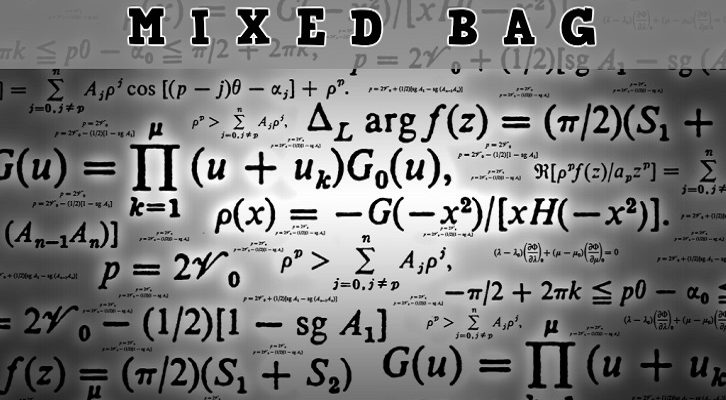# Solutions to CAT Geometry Data Sufficiency Questions

Have given below the solutions to the questions on geometry DS. The solutions are courtesy Vimal Gopinath (person incharge of 2IIM Bengaluru)

Qn 1: Is triangle ABC obtuse angled?

I) a^2 + b^2 > c^2 – Not enough. We don’t have info about b^2 or a^2

II) The circumcenter of the triangle does not lie inside the triangle – Not enough. The triangle could be right-angled as well.

Combination is also not enough, it is valid for both right angled and obtuse angled triangles.

D

Qn 2: Do the two circles with centers A and B and radii R and r intersect each other

I) AB > R – r – Not enough. May intersect, may be “parallel” or “disjoint”

II) AB > R + r – Sufficient. Circles cant intersect. Have to be separate.

A

Qn 3: Trapezium ABCD is such that AB is parallel to CD. Is this trapezium anisosceles trapezium?

I) Angle B and D are supplementary Sufficient. If two base angles are equal, then the trapezium has to be isosceles.

II) The quadrilateral is inscribed inside a circle. Sufficient. All trapeziums inscribed in circles have to be isosceles. (Think about the proof for this)

C

Qn 4: Circle C has center O, and a chord AB such that angle AOB = 80 degrees.Does point E lie inside the circle

I) Angle AEB > 50 degrees Insufficient.E could lie on the minor segment ADB or slightly outside or slightly inside the circle.

II) Angle AEB < 30 degrees. Sufficient. All the angles inside the circle will be in the range from 40 – 140 degrees. Anything less than 40 will have to be outside the circle.)

A

### Related posts

1.maniac says:
2.Bee says: